## Introduction

The development of efficient, stable, and accurate models that account for wheel–rail contact is of great interest for vehicle developers in the industry, for vehicle operators and infrastructure managers and also for researchers. The railway system is a complex one, with strong and subtle interactions between the different components of the vehicle, within the track system, and in the wheel/rail interface. This complexity is addressed by vehicle dynamics simulation, providing a well-established tool for the design and analysis of many aspects of railway systems, such as ride comfort, running stability and safety, virtual homologation, and the prediction of wear and rolling contact fatigue [1,2,3,4,5,6,7]. Wheel–rail interaction is a key aspect in these simulations: using steel-on-steel contacts, very high forces are transmitted in small areas, which may change quickly with the contact circumstances, such as the contact location, creepage, and the available friction. This leads to the problem of wheel/rail contact modelling for multibody system simulation.

The multibody modelling approach sets a framework, where a model consists (typically) of discrete elements like rigid bodies and force elements. Force elements are then connected to the bodies at markers, i.e. at special points where the kinematics on the one hand and the forces and moments on the other hand are exchanged. Within this context, wheel–rail contacts are usually modelled as a specific type of force element. This receives the kinematical quantities (translational and rotational displacements and velocities) from the markers, at which it is connected to the bodies representing the wheel and the rail. The force element provides an effective means for modular programming, permitting the contact problem to be solved in isolation, treated as a black-box from the side of multibody dynamics.

In the implementation of a force element for multibody system dynamics, the wheel–rail contact problem is generally divided into three consecutive stages [2, 8,9,10,11,12]: (1) the contact geometry stage, identifying the contact location and corresponding tangent plane; (2) the kinematic stage, determining the undeformed distance and the creepages, i.e. the relative motion of wheel and rail surfaces, at the contact position; and (3) the contact mechanics stage, computing the resultant normal and tangential forces. The background lies in the physical phenomena involved in wheel–rail contact, as schematized in Fig. 1. These phenomena differ widely between normal and tangential directions, introducing a strong anisotropy in the contact problem . The third stage is divided further into two sub-stages, for the normal and tangential contact solution. For instance combining a Hertzian approach for the normal contact with Fastsim or Polach’s method for the tangential problem [14, 15].

The tangent plane provides a convenient interface between the quantities and coordinates of the multibody system approach, on one side, and of rolling contact mechanics, on the other. It provides the coordinate directions needed to define the contact problem: (a) the so-called undeformed distance function, for the normal contact problem, and (b) the rigid slip velocity, i.e. surfaces’ relative motion, for the tangential one. These directions are used next to express the solution: (c) the contact pressures, acting in normal direction, and (d) frictional stresses, acting tangentially. That is, the tangent plane provides a further means for modular programming, partitioning the wheel/rail contact into the preparations and post-processing, versus the actual solution. The actual solution is then performed in isolation, in terms of coordinates that are natural for the normal and tangential contact problems.

However, the tangent plane does not allow one to capture contact problems fully. The method runs into difficulties for asymmetric, non-Hertzian problems, where the tangent plane can be defined in different ways, with different inclinations [16,17,18]. Which one represents the true contact interactions in the best way? Further complications arise in two-point contact situations, where the interaction between the two problems needs to be accounted for [19, 20], and where discontinuities occur when the main contact jumps from one point to the other , and in conformal contacts, where the curvature of the contact patch is inherent in the problem specification [22,23,24,25]. How can the true gap and rigid slip best be mapped from the curved surfaces onto a single plane?

Here we present the “curved reference surface”, called curved reference for short, as an alternative backbone for the wheel/rail contact solution. This builds on the generalised curvilinear coordinates $$[x,s,n]^{T}$$ that were introduced for conformal contact situations [22, 23]. We show how these ideas are worked out in the automated analysis of the contact geometry problem in CONTACT. A key element is to use a form of object orientation, using “markers” to capture positions and orientations precisely, and “grids” to manipulate with curves and surfaces, with spline interpolation . This software foundation is extended for the curved reference and curved contact surface. Next, the undeformed distance is computed relative to the local normal direction. The rigid slip velocity is computed separately at each potential contact position, by-passing the creepages that simplify the actual situation on the basis of a reference position that is open to question. Curvature of the contact surface in rolling direction is incorporated using a so-called “mid-plane correction”, to make the rigid slip behave in the desired way, avoiding rigid slip at places where it is not expected. The contact problem is solved in the curved coordinate system, after which the resulting tractions are rotated and aggregated to the resultant total forces and moments.

The method using a curved reference surface provides a natural extension of the elastic approach as discussed by Shabana et al. , illustrated in Fig. 2 (b), which placed strong emphasis on the contact location and the contact forces as the primary concepts in the calculation. Acknowledging that contact arises on a contact area with finite extensions, a distributed force element may be considered for the solution of the contact problem. This lets us account for the local, distributed kinematics of the problem rather than using approximate values obtained from a single contact location, and defines the actions on the wheel and rail by the contact stresses, as shown in Fig. 2 (c), rather than using the contact forces as the central concept. The curved reference provides a means to approximate this real contact configuration. The roles of the contact location and contact forces are much reduced; these are used in the software implementation, as a means to summarize the situation in a convenient way, as needed by the multibody simulation. Different from the original elastic approach, the contact point may then be selected after the contact solution, and does not affect the solution anymore.

The content of this paper is structured as follows. First, Sect. 2 gives a concise description of rolling contact problems. This shows the planar contact approach embedded in the wider mathematical context and indicates the places where extensions are made for the curved reference. The extensions are discussed in Sect. 3: presenting the overall structure of the computations and the detailed choices made in the proposed method. Numerical results are presented in Sect. 4, applying the new method to two-point and conformal contacts, and as a reference for benchmarking planar contact approaches. After this, the paper ends with conclusions and discussion.

## Formulation of the contact problem

Here we describe the mathematical formulation of rolling contact problems, laying the foundations for the extensions presented in consecutive sections. We start from the viewpoint of a dynamical system, to show the embedding of contacts in a multibody formulation. This aims to give a better understanding of the undeformed distance and rigid slip functions, i.e. the quantities that are at the heart of the contact problem. These are related to the bodies’ positions and deformations as seen from the multibody perspective.

Two coordinate systems are used: $$[x,y,z]^{T}$$ for a global system, with $$z$$ the overall vertical direction normal to the track plane, positive downwards, and $$[x,s,n]^{T}$$ for local coordinates used to formulate the contact problem.

### Undeformed reference state

We consider two elastic bodies, particularly a rail and a wheel, that undergo rolling with sliding motion. They are indicated by numbers $$a$$, with $$a=1$$ for the rail, the upper body ($$z$$ positive downwards), and $$a=2$$ for the wheel. Following the Arbitrary Lagrangian Eulerian (ALE) descriptions given by Nackenhorst  and Donea et al. , we first identify the geometries of the bodies separately, without being in contact, named the initial state. Particles of the bodies are referred to by their coordinates $$\mathbf{X}$$ in the coordinate system that is used, with $$\mathbf{X} \in \Omega _{ini}^{(a)}$$, where the domain $$\Omega _{ini}^{(a)}$$ represents body $$a$$ in its initial (undeformed) state.

Next, the bodies are brought together such that contact is formed. Here, too, we identify the geometry without considering deformations, the so-called undeformed reference state. This can be formalized using mappings $$\boldsymbol{\Psi }^{(a)}$$ for bodies $$a=1,2$$ that describes the reference position,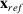, of each particle $$\mathbf{X}$$ at the current time instance $$t$$.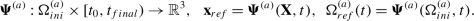(1)

These mappings are used to describe rigid body translation and rotation. An example concerns a wheelset that is moved from an arbitrary initial position to the current position $$\mathbf{x} _{ws}$$ and orientation $$\boldsymbol{\nu }_{ws}$$ (Euler angles). The reference position of each particle is obtained as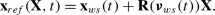(2)

Here $$\mathbf{X}$$ is the position of a particle relative to the center of mass and $$\mathbf{R}$$ is the $$3\times 3$$ rotation matrix corresponding to the orientation relative to the initial one.

Considering flexible multibody dynamics, the overall flexible bending of rails, axles, wheels, occurs at a much slower rate than the elastic deformation in and around the contact areas. “Global” deformations are therefore kept separate from the “local” contact solution. This is formalized by incorporating global deformations in the reference state. The mapping could be decomposed into parts, separating rigid body motion from flexible bending, as used in . Here we focus on the combined result of these different motions.

### Elastic displacements

The third state that is considered is the actual configuration at time $$t$$, including (local) deformation. This is described by further mappings $$\boldsymbol{\Phi }^{(a)}$$.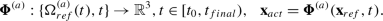(3)

This allows one to introduce local elastic displacements of all particles as the difference between actual and reference states: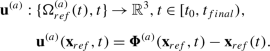(4)

The particles’ velocities are obtained as the material time derivative of their actual positions. By Eq. (4), this may be split as follows: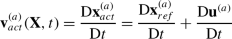(5)

The reference part comes from rigid body motion and (slow) overall flexible bending, which are assumed known in the solution of contact problems. The other part describes the change of elastic displacements of particles due to the contact. These are an important concern in frictional contacts with rolling and sliding.

### Considerations on the normal direction

The bodies are brought into contact and, as a result, stresses $$\boldsymbol{\sigma }$$ (tensor), strains $$\boldsymbol{\epsilon }$$ (tensor) and displacements $$\mathbf{u}$$ (vector) arise in the bodies and at their surfaces.

The usual boundary conditions for a single body are to prescribe displacements $$\mathbf{u} ^{(a)}=\bar{ \mathbf{u} }^{(a)}$$ on one part of the boundary $$\Gamma _{u}^{(a)}\subset \partial \Omega ^{(a)}$$ and stresses $$\boldsymbol{\sigma }^{(a)} \mathbf{n} ^{(a)}=\bar{ \mathbf{p} }^{(a)}$$ at another part $$\Gamma _{\sigma }^{(a)}\subset \partial \Omega ^{(a)}$$. This does not work at contacts because the two bodies form each others boundary conditions. Neither $$\bar{ \mathbf{u} }$$ nor $$\bar{ \mathbf{p} }$$ is known, so that these cannot be prescribed in advance.

In the study of contact mechanics, the stresses $$\bar{ \mathbf{p} }$$ acting at the outside of a continuous, deformable body, are often called “tractions” or “surface tractions” [13, 31]. That is, pressures are also called “normal tractions”, and what are called “tractions” in other fields of study are here referred to as “tangential tractions”. This stems from the central role of surface stresses in contact interactions, by which it is convenient to have a short term for their designation.

Contact conditions are introduced to complete the specification of the problem of the bodies’ deformation. This starts from regions $$\Gamma _{c}^{(a)}\subset \partial \Omega ^{(a)}$$ that include all points on the two bodies that may get in contact. One body may then be designated as the master body, using its local surface normals $$\mathbf{n} ^{(a)}( \mathbf{x} )$$ to identify corresponding points on the other body. Having established such a relation, the so-called gap function can be defined, as well as the mutual velocity of the two points.

The main difficulties of such a scheme become clear from an example, considering two soft equal spheres that are pressed together. A flat contact area is formed that may be relatively large compared to the size of the spheres. In this example, large deformations occur that introduce considerable differences between the surface normals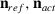in reference and actual configurations. This complicates the formalization of the gap function: should this be measured in the initial or in the final direction? Furthermore, this also complicates the mapping of the two surfaces onto each other. How can we locate the corresponding point on the other body if the surface normals change considerably by deformation?

Generic solutions to these problems have been established; see for instance [32,33,34] for strategies used in finite-element methods. Instead, we require that the normal and tangential directions are defined a priori to the contact solution: identical for all surface positions when using a planar contact approach, or space-varying when using the conformal method.

### Introducing a curved reference surface

In formulating the contact problem, we are particularly interested in the surface quantities. According to the anisotropy of contact problems (Fig. 1), this needs the local normal and tangential directions. But the actual surface normals, after deformation, become known only after the contact solution. This then calls for iteration, building the solution in progressively finer detail, or approximation, ignoring certain effects on the surface normals.

Assuming that elastic displacements and displacement gradients are small, the surface normals cannot change much between reference and actual configurations [35, p. 3]. This allows one to use the surface normals of the reference configuration as a basis for the contact solution. Further, if the contact area is small compared to the local dimensions of the contacting bodies, that is, if the contact is concentrated, the contact will be effectively flat, contained in a plane, permitting a planar approximation. This latter assumption will now be relaxed, forming a curved reference surface on the basis of the undeformed configuration.

A curved reference surface is introduced in between the wheel and rail surfaces, with surface parameters $$s_{1}$$ and $$s_{2}$$ (cf. ):

$$\mathbf{x} _{cref} : \mathcal{S} \rightarrow \mathbb{R}^{3}, \mathcal{S} \subset \mathbb{R}^{2}, \;\; \mathbf{x} _{cref} = \mathbf{x} _{cref}(s_{1},s_{2}) .$$
(6)

At each position $$\mathbf{x} _{cref}$$, the tangent directions are found using

$$\mathbf{x} _{,1} = \frac{\partial \mathbf{x} _{cref}(s_{1},s_{2})}{\partial s_{1}} , \;\; \mathbf{x} _{,2} = \frac{\partial \mathbf{x} _{cref}(s_{1},s_{2})}{\partial s_{2}} ,$$
(7)

and the local normal to the surface is

$$\mathbf{n} (s_{1},s_{2}) = \frac{ \mathbf{x} _{,1} \times \mathbf{x} _{,2}}{| \mathbf{x} _{,1} \times \mathbf{x} _{,2}|} .$$
(8)

It is assumed that the curved reference lies close to the surface where the actual contact occurs, and that its normal vector $$\mathbf{n}$$ is close to the surface normals of rail and wheel, before or after deformation. The curved reference is then used in place of the surface in which the actual contact area is contained, and the normal vector $$\mathbf{n}$$ of Eq. (8) is used instead of the surface normals of rail and wheel. The method may be applied with different strategies for the construction of the curved reference. The better this approaches the actual contact, the smaller will be the errors of approximation.

Next, the boundaries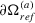are projected onto the curved reference surface. This gives $$(s_{1},s_{2})$$-coordinates for each boundary point on the wheel and the rail. This mapping can be inverted in the contact area and its surroundings, by which each $$(s_{1},s_{2})$$ maps to a single point on the rail and wheel surfaces.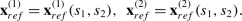(9)

By this projection, quantities like the displacements $$\mathbf{u} ^{(a)}$$ or the tractions $$\mathbf{p} ^{(a)}$$ that are defined on the actual surfaces may be considered as functions defined on the curved reference surface instead.

### Contact conditions

We consider the positions and velocities for two particles that are in contact with each other in the actual configuration. Firstly, their distance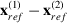in the reference state prescribes the difference of their elastic displacements $$\mathbf{u} := \mathbf{u} ^{(1)}- \mathbf{u} ^{(2)}$$ in the actual state. This yields a constraint in the normal direction, concerning the gap or undeformed distance of the profiles, with negative values for interpenetration.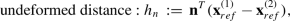(10a)
\begin{aligned} \mbox{deformed distance} :& e_{n} \;:=\; \mathbf{n} ^{T} ( \mathbf{x} _{act}^{(1)}- \mathbf{x} _{act}^{(2)}) \;\;=\;\; h_{n} + \mathbf{n} ^{T} \mathbf{u} . \end{aligned}
(10b)

Concerning the velocities, we may measure and compare the velocity differences in the reference and actual states. These are called the rigid slip velocity and actual slip velocity, denoted by $$\mathbf{w} , \mathbf{s}$$. Here we are interested primarily in the components in tangential directions: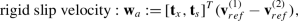(11a)
\begin{aligned} \mbox{actual slip velocity} :& {} \mathbf{s} _{a} := [ \mathbf{t} _{x}, \mathbf{t} _{s} ]^{T} ( \mathbf{v} _{act}^{(1)} - \mathbf{v} _{act}^{(2)} ) \;=\; \mathbf{w} _{a} + [ \mathbf{t} _{x}, \mathbf{t} _{s}]^{T} \frac{\mathrm{D} \mathbf{u} }{\mathrm{D}t} . \end{aligned}
(11b)

A subscript $$[]_{a}$$ is added to indicate that these are absolute dimensioned values (e.g. $$[\mbox{mm}/\mbox{s}]$$) rather than relative dimensionless values ($$[-], [\%]$$). Equation (11b) may be scaled by an overall rolling velocity $$V$$, replacing absolute slips by relative slips $$\mathbf{w} _{r}, \mathbf{s} _{r}$$. This brings in that many rolling contacts are insensitive to the velocity $$V$$, producing the same tractions as long as the same relative rigid slip is maintained. This is mostly a matter of presentation, the same solutions may be computed with or without such scaling.

Using these preparations, the contact conditions may be stated in their customary form [31, 35, 36]:

\begin{aligned} &\mbox{normal problem} : \\ &\mbox{ in exterior area E} : \hspace{25pt}e > 0, \; p_{n} = 0, \end{aligned}
(12a)
\begin{aligned} &\mbox{ in contact C=H\cup S} : \hspace{10pt} e = 0, \; p_{n} \ge 0, \end{aligned}
(12b)
\begin{aligned} &\mbox{tangential problem} : \\ &\mbox{ in exterior area E} : \hspace{25.2pt} \mathbf{s} _{a} \mbox{ free}, \; \mathbf{p} _{t} = \mathbf{0} , \end{aligned}
(12c)
\begin{aligned} &\mbox{ in adhesion area H} : \hspace{18.8pt} \| \mathbf{s} _{a}\| = 0, \; \| \mathbf{p} _{t}\| \le \mu p_{n}, \end{aligned}
(12d)
\begin{aligned} &\mbox{ in slip area S} : \hspace{42pt} \| \mathbf{s} _{a}\| > 0, \; \mathbf{p} _{t} = -\mu p_{n}\, \mathbf{s} _{a} / \| \mathbf{s} _{a} \|. \end{aligned}
(12e)

Here $$\mathbf{p} = \mathbf{p} ^{(1)}$$ stands for the surface tractions acting on body 1, $$\mathbf{p} ^{(2)}=- \mathbf{p}$$, with $$p_{n}$$ the component in (local) normal direction and $$\mathbf{p} _{t}=[p_{x}, p_{s}]^{T}$$ the tangential parts. Equations (12a) and (12b) state that the normal pressure is compressive and that there is no interpenetration between the bodies. Equations (12d) and (12e) correspond to Coulomb’s law of dry friction. That is, the tangential tractions cannot exceed the local traction bound $$\mu p_{n}$$, slip occurs only where the traction bound is reached, and if there is slip, the tractions act in the opposite direction.

The coefficient of friction $$\mu$$ is often considered as a known input to the simulation, optionally with independent values at the top and side of the rail. The values used depend on circumstances like contamination (e.g. wear debris, clay, leaves) and applied agents such as friction modifiers and flange lubrication. On-going research is considering whether this model suffices and if so, how $$\mu$$ comes about, modeling the effects of third body layers, roughness, fluids, temperature, etc. on the creep forces [37,38,39,40,41,42].

### Planar contact approach

Three more steps remain to complete the elastic, planar contact approach for contacts in rail vehicle dynamics simulation: (1) defining the tangent plane for the computations, i.e. filling in the normal direction, (2) defining the creepages, thereby specifying the rigid slip, and (3) solution or approximation of the resulting, coupled elasticity problems. The extension to conformal contacts is presented in Sect. 3.

The identification of an appropriate tangent plane was discussed in previous publications [17, 18, 26]. This begins with an analysis of the wheel and rail geometries in their undeformed states, locating the regions where contact may occur. For each region one can look at the so-called initial contact position, the point with maximum interpenetration, and define the contact plane tangent to the surfaces at this position. This is the simplest initial contact method. One extension is to set the inclination for the tangent plane in some averaged way. Another alternative is to base the tangent plane off a different reference location, typically using some form of weighted averaging [16, 26]. This is called the weighted center method.

Weighted averaging can be worked out in different ways: using the horizontal interpenetration area, the vertical interpenetration area at the contact locus, the 3d interpenetration volume, using estimated contact pressures, and so on. Here we employ weighting using linear or quadratic functions of the vertical gap values $$g(x,y)$$ as shown in Eqs. (13) and (14) ,

\begin{aligned}{} [ x , y, \alpha ]_{cwgt1} = \iint _{g< 0} [x, y, \alpha ] \, g(x,y) \; dx \,dy \; \Big/ \iint _{g< 0} g(x,y) \; dx\,dy , \end{aligned}
(13)
\begin{aligned}{} [ x , y, \alpha ]_{cwgt2} = \iint _{g< 0} [x, y, \alpha ] \, g^{2}(x,y) \; dx\,dy \; \Big/ \iint _{g< 0} g^{2}(x,y) \; dx\,dy . \end{aligned}
(14)

The different methods composed from this are defined in Table 1. The motivation for using squared gaps is that they concentrate more on the bigger gap values. This appears to correlate better to the contact pressures than the linear gap values.

The rigid slip velocity $$\mathbf{w} _{a}$$ of Eq. (11a) is a field that describes the tangential velocity difference of the two surfaces at every point in contact. This field is usually simplified, considering the velocity difference at “the” contact location. Three creepages $$\xi ,\eta ,\phi$$ are then defined for longitudinal, lateral, and spin motions. Longitudinal and lateral creepages are found as the scaled velocity difference at the contact position, while spin creepage represents the scaled relative rotation about “the” normal direction. Generic formulas may be derived from the velocity of points on rigid bodies, using the time derivative of Eq. (2); see for instance [11, 12] or . These formulas may also be expanded using the wheelset dimensions and orientation, to get creepages in terms of railway-specific terms, e.g. [43,44,45].

When using creepages, the scaled rigid slip velocity is presented in a compact, yet approximate way, i.e.

$$\mathbf{w} _{r}( \mathbf{x} ) = \left [ \textstyle\begin{array}{c} \xi - \phi \, s \\ \eta + \phi \, x \end{array}\displaystyle \right ] .$$
(15)

Here $$\mathbf{x} =[x,s]^{T}$$ is a position in the tangent plane, defined relative to the local origin used, i.e. the contact reference position . A complete derivation was presented by Kalker [31, §1.7.3]. This shows that small terms are neglected compared to the complete rigid slip of Eq. (11a).

Historically, the notion of rigid slip did not appear until the end of the 1970-ies. No symbol was used for this in older work by Kalker, writing out formulas like $$s_{x}=\nu _{x}-\phi y+ \partial u/\partial x$$ [46, Eq. (1.6a)], using $$\nu _{x}$$ instead of $$\xi$$. This notation was maintained by Johnson in 1985, e.g. writing $$\xi _{x}-\psi y/c$$ in [47, Eq. (8.3a)]. This work used the symbol $$\mathbf{s}$$ for the actual slip.

The term rigid slip seems to have been introduced with the variational theory for rolling contacts [48, 49]. This placed more emphasis on the (undeformed) reference state and its elastic deformation, and for rolling, on the motion of separate particles instead of the bodies as a whole. New symbols were introduced, inconsistently, denoting the rigid and actual slips as $$\mathbf{r} , \mathbf{s}$$ in , while using $$\mathbf{s} , \mathbf{w}$$ in . The background of the latter is found in a paper in German , where rigid slip is translated as “starre Schlupf$$\mathbf{s}$$ and actual slip as “wahre Schlupf$$\mathbf{w}$$. Kalker used this notation up to about the paper introducing Fastsim . The roles of $$\mathbf{s}$$ and $$\mathbf{w}$$ have been interchanged thereafter, denoting the rigid slip as $$\mathbf{w}$$, as followed here in this paper.

### Elasticity in the contact problem

The description of the contact mechanics problem is completed by presenting the laws for the material behavior. Adopting small displacements theory, these are generically described as

\begin{aligned} & \rho ^{(a)} \ddot{ \mathbf{u} }^{(a)} = \nabla \cdot \boldsymbol{\sigma }^{(a)} + \mathbf{f} ^{(a)} , \end{aligned}
(16a)
\begin{aligned} & \boldsymbol{\sigma }^{(a)} = \mathsf {C}^{(a)} : \boldsymbol{\epsilon }^{(a)} , \end{aligned}
(16b)
\begin{aligned} & \boldsymbol{\epsilon }^{(a)} = \frac{1}{2} \left [ \nabla \mathbf{u} ^{(a)} + (\nabla \mathbf{u} ^{(a)})^{T} \right ] . \end{aligned}
(16c)

Here $$\rho ^{(a)}$$ is the mass density, $$\mathbf{f} ^{(a)}$$ represents body forces, and $$\mathsf {C}^{(a)}$$ is a fourth-order stiffness tensor. Equation (16a) is the equation of motion (Newton), Eq. (16b) describes the material behavior according to Hooke’s law, and Eq. (16c) is the linearised strain–displacement relation.

These equations are assumed to hold everywhere in the bodies’ interiors $$\mathbf{x} _{act}\in \Omega _{act}^{(a)}$$, where suitable boundary conditions are required on the boundaries $$\partial \Omega ^{(a)}$$. Considering a single body, this gives rise to initial and boundary value problems, amenable to finite-element solution. In-depth information is provided by Laursen , for instance, including the extensions to large deformations and to include contacts into the formulation.

Instead of approaching the elasticity equations (16a)–(16c) directly, using finite elements, it pays off to first reformulate the problem. One main approach for this is to use a boundary element method. This method is based on the concept of influence functions $$\mathbf{A} ( \mathbf{x} , \mathbf{x} ')$$, which establish a relation between the displacements $$\mathbf{u}$$ at surface position $$\mathbf{x} =[x,s,0]^{T}$$ and a tractions $$\mathbf{p} ( \mathbf{x} ')$$ at another surface position $$\mathbf{x} '= [x',s',0]^{T}$$. For linearly elastic behavior, the full solution $$\mathbf{u}$$ can then be composed by superposition:

$$\mathbf{u} ^{(a)}( \mathbf{x} ) = \int _{ \mathbf{x} '\in C} \mathbf{A} ^{(a)}( \mathbf{x} , \mathbf{x} ') \mathbf{p} ^{(a)}( \mathbf{x} ') \, dx'\,ds'$$
(17)

This method considers stresses and deformations at the surfaces only, avoiding the discretization of the interiors of wheel and rail bodies.

The influence functions depend on the geometry and material parameters of the two bodies. They can be computed numerically and stored in a file [22, 25], or can be calculated analytically in certain specific cases. The latter is particularly convenient for concentrated contact problems that permit using the halfspace approach. Considering homogeneous, isotropic, linearly elastic materials, and neglecting inertial effects, which are assumed to be small compared to the contact stresses , the influence functions can be calculated exactly from the formulas of Boussinesq and Cerruti [35, 55].

The equations are discretized in CONTACT using a rectangular potential contact area $$\Gamma _{c}$$ that needs to encompass the actual contact area $$C$$, discretized into $$m_{x}\times m_{s}$$ elements of size $$\delta _{x}\times \delta _{s}$$. Subscripts $$i,j$$ are used for grid point numbers in $$x$$- and $$s$$-directions. For the sake of simplicity, the indices $$i,j$$ are merged into a single index $$I=i+m_{x}\cdot (j-1)$$.

The continuous tractions $$\mathbf{p}$$ are approximated by piecewise constant or piecewise bilinear functions . Purpose-built solvers were constructed for the resulting numerical problems, i.e. NormCG for the normal problem  and TangCG, SteadyGS [57, 58] for the tangential problem. These are based on Conjugate Gradients and the Gauss–Seidel method, extended with active set strategies for dealing with the contact conditions (12a)–(12e), and using FFTs for fast computation of structured matrix-vector products.

### Heuristic approaches

The boundary element approach implemented in CONTACT yields a complete, “exact” treatment of wheel–rail contacts at sufficiently flat sections the wheel and the rail. With the increasing speed of computers, this can now be integrated in multibody dynamics simulation, as shown by recent publications [42, 59, 60]. This provides benefits in a number of special situations where a high level of detail is needed, such as computations of locomotive traction power including the effects of third body layers, flange-climb derailment with a conformal geometry, wheel-flats or corrugation, studies of wear and RCF damage that need detailed contact stresses, or validation of more simplified contact approaches.

However, even though CONTACT may be thousands of times faster than using the finite-element method, it remains rather slow for vehicle dynamics simulations considering longer stretches of track, where millions or billions of contact problems must be solved. Fast approximations are therefore preferred for day-to-day work, in most cases, as long as one is not too critical on the results.

Many methods have been presented for the modelling of the contact forces [14, 15], which work by simplifying the full contact model in one way or another. Normal contact was analyzed by Hertz in a seminal paper , providing a detailed solution for frictionless contacts for surfaces that have constant curvatures in the vicinity of the contact [31, 47, 62]. Under these assumptions, an elliptical contact arises with a semi-ellipsoidal pressure distribution. This has long served as a reasonable approximation in railway vehicle dynamics simulation, and is provided still in several simulation packages today. One main reason is that the Hertzian approximation brings the tangential contact problem into a standard form, simplifying its approximation .

Since real wheels and rails have varying curvatures along the width of the profiles, there has been much work aiming to extend the normal contact to non-Hertzian geometries and non-elliptical contact patches. Heuristic methods were proposed using virtual interpenetration, multi-Hertzian and semi-Hertzian strategies [9, 15, 16, 20, 63,64,65,66]. These generally work by assuming a correspondence between the actual contact area and the interpenetration area, and a pressure distribution analogous to the Hertizan pressures. Iterative solution of the elasticity equations, as done by NormCG, is thereby avoided and replaced by a much reduced set of equations, dependent on the heuristic that is used. Various extensions were published in recent years; see e.g. [12, 67,68,69,70], addressing for instance the effect of a non-zero yaw angle. More work needs to be done on how good these methods perform, in terms of speed and accuracy, in a wide range of circumstances.

The tangential creepage and creep force are related to each other in a complex, non-linear way. In vehicle dynamics simulation, this is approached with different heuristics by Vermeulen and Johnson , Shen–Hedrick–Elkins , Polach [73, 74], Bosso et al. , or by using Fastsim [36, 53], or by building a table on the basis of CONTACT’s results . Most of this work is based on an assumption of an elliptical contact. Solution of the elasticity equations is avoided by adopting a formula, based on certain theoretical considerations (Vermeulen–Johnson, Shen–Hedrick–Elkins, Bosso et al.), or by adopting a simplified material law instead of Eq. (16a)–(16c) (Fastsim), or by analytical approximation of the results of the Fastsim approach (Polach).

Only few works considered the creep forces in non-Hertzian conditions. An extension of Fastsim is provided by the FaStrip algorithm , but this does not seem to be picked up widely. The table-based approach is also extended to non-Hertzian circumstances [78, 79]. Fastsim itself can be employed if a strategy is defined to get the flexibilities needed. The main strategy here is using an equivalent ellipse , an alternative route is presented by Alonso and Giménez . Further work is needed to develop and test algorithms for non-Hertzian circumstances.

## Extensions for conformal contacts

This paper presents the extension of the above approach for planar contact by combination with the ideas for conformal contacts as presented in [22, 23]. This revolves around the added value provided by the generalized curvilinear coordinates $$[x,s,n]^{T}$$ that are extended here to the curved reference surface. These coordinates replace the Cartesian contact coordinates $$[x_{cp},y_{cp},z_{cp}]^{T}$$. The curved reference replaces the tangent plane as the central backbone for the computations, decoupling actual contact problem from the preparations and post-processing, and decoupling the normal from the tangential contact solution. The computations are generalized further to the 3-d contact search procedure as presented in  and are automated using the mechanisms presented in there.

Section 3.1 presents the overall solution approach. Section 3.2 introduces the curved reference, while Sect. 3.3 describes its computation. The extension of undeformed distance calculations and rigid slip velocities are presented in Sects. 3.4, 3.5 and 3.6, the latter introducing a new mid-plane correction. Section 3.7 finally addresses the aggregation of resulting forces and moments.

### Overall solution procedure

In a separate paper , we presented the analysis of contact geometry using the planar contact approach. This is built on an object oriented-like software foundation for markers, grids and coordinate transformations. The main steps of the method are summarized in Fig. 3, with steps 0.b to 2.a illustrated graphically in Fig. 4.

The method starts by reading wheel and rail profiles from various sources, within their own coordinate systems, making corrections and preparations to bring them into a canonical form. Figure 4 (a) displays placing of these profiles in a common coordinate system, the track frame $$(tr)$$, using markers $$\mathbf{m} _{rw(tr)}$$ and $$\mathbf{m} _{rr(tr)}$$ that connect the profiles to the bodies representing the wheel and the rail, governing their kinematics. This uses geometrical and kinematical information from a half wheelset on a half track or a half pair of rollers. The rail is considered prismatic in rolling direction, whereas wheels and rollers are assumed bodies of revolution. Track irregularities and rail and wheelset flexibilities are included as provided by the multibody model.

The location of contact patches is determined using the interpenetration in overall vertical direction (Fig. 4 (b)). A full 3-d contact search is performed including effects of the yaw angle, building the wheel surface in wheel coordinates first, then transformed to the track system. Interpenetration areas can be determined using a brute force grid-based approach or using a refined version of the contact locus . Interpenetration areas are described by the interval $$[y_{sta},y_{end}]$$ in Fig. 4 (b) and by the length $$[x_{sta},x_{end}]$$ (not shown). These may be larger but not smaller than the actual interpenetration area, as required by the potential contact area. In the software, the name “contact patch” is used as a synonym for “independent contact problem”, storing all corresponding information.

The contact reference position and reference angle are computed using one of the approaches of Sect. 2.6. This yields the contact marker $$\mathbf{m} _{cp(tr)}$$ and contact plane as illustrated in Fig. 4 (c). The interval $$[y_{sta},y_{end}]$$ is projected onto this plane to get the corresponding extent $$[s_{sta},s_{end}]$$ in the contact coordinate system. Adjacent contact patches may be taken together when the distance between them is less than a threshold, using a blending approach to mitigate jumps in the numerical results at perturbation of the input values . From there, the discretization is formed, the undeformed distance and creepages are computed on each contact patch (Fig. 4 (d)), and the contact problems are solved using the halfspace approach, using the discretization and solvers as discussed in Sect. 2.7.

### Introducing the curved reference surface

A curved reference surface is now introduced for conformal contacts as illustrated in Fig. 5, using the curved coordinate $$s$$ in the tangential direction and the curved coordinate $$n$$ in the normal direction. A main characteristic of this reference surface is that it uses a local construction, producing separate curved reference grids for contact patches that are computed separately, instead of one curved reference for all contact patches together. The reason for this is illustrated in Fig. 6: the construction of a single, global curved reference is complicated for geometries that have multiple, unconnected interpenetration zones with bigger positive gaps in between. In such cases we cannot maintain correspondence between arc lengths $$\tilde{s}, s_{r}, s_{w}$$ of the different surfaces, requiring a more complex administration.

A second characteristic of our curved reference is that we consider the curvature in lateral direction only. Actual contact surfaces can be curved in longitudinal direction too, for instance a raceway in a ball bearing, or for a pin rolling in a hole. This curvature in longitudinal direction affects the rigid slip in wheel–rail contacts, as will be explained more in detail below. Yet to consider this would make our software more complicated. A prismatic curved surface can be represented by a 1-d grid (curve) in the $$Oyz$$-plane, and the transformation to Cartesian coordinates involves just a single rotation. This makes intermediate and final results of the program easier to understand and work with in further computations.

A beneficial result of using locally defined curved references is that they fit nicely in the overall structure as shown in Fig. 3. The steps for the planar approach are all reused, with relatively minor extensions to accommodate conformal contacts. The steps are modified as follows:

1.c’:

The potential contact area is defined on the curved reference surface.

2.a’:

The interpenetration of the undeformed surfaces is measured in the local normal direction of the reference surface, i.e. using the local orientation of the normal instead of a constant normal direction defined for the whole contact area;

2.b’:

The apparent slipping of the undeformed surfaces (rigid slip) is measured along the local tangent vectors instead of using a single tangent plane for the entire contact area;

3.a’, 3.b’:

The true elastic deformations of the wheel and rail can be modelled using influence functions for non-planar bodies, e.g. computed by finite-element methods, instead of using the half-space approximation. This extension is skipped here. The development of influence functions is discussed in [81, 82].

3.c’:

The surface tractions are defined using the local normal and tangent directions, and this is accounted for in the accumulation of the total forces and moments.

### Step 1.c’, Defining the curved contact surface

The curved reference serves as the potential contact area for the contact solution. Using rectangular discretization elements, this surface should have fixed grid sizes $$\delta x$$ and $$\delta s$$, with coordinates $$\mathbf{x} _{I}$$ following the curved rail and wheel surfaces. Using the rail as the basis for the coordinate system, this grid will be defined by the positions $$\{ x_{i} \} \times \{y_{j},z_{j} \}$$. Corresponding information can then be stored using 1-d grids and grid-functions as defined in [26, §2.6].

A simple approach for the curved reference would be to simply follow the rail profile. This may work well as long as the wheel and rail profiles stay in close proximity to each other, but introduces slight deviations in the surface normals. This is illustrated in Fig. 7 for circular profiles. Note that the left figure shows an extreme situation, with a contact width of $$5~\mbox{mm}$$ on a ball diameter of $$20~\mbox{mm}$$. For steel bodies, such a contact width may be realistic only if the rail profile is grooved much stronger. In that case the surface normals will stay much closer together, as shown in the right part of the figure.

A more refined approach is used that is based on the dominant effects in the elastic deformations. For equal material laws and equal material parameters, the actual contact lies halfway between the undeformed wheel and rail, and the surface normal is averaged as well. This ignores the effect of the geometry of the solids on the deformations, which is also ignored when using the halfspace approach.

In case of material dissimilarity, the notion “in between” the two surfaces may be refined using appropriate scaling. The direct effect of pressures $$p_{n}$$ on normal displacements scales with $$(1-\nu )/G$$. This is incorporated in the mean surface using

\begin{aligned} {} \mathbf{x} _{m} =& \theta ^{(1)} \mathbf{x} ^{(1)} + (1-\theta ^{(1)}) \mathbf{x} ^{(2)}, \;\mbox{with} \end{aligned}
(18a)
\begin{aligned} \theta ^{(1)} =& \frac{ (1-\nu ^{(1)}) G^{(2)} }{ (1-\nu ^{(1)}) G^{(2)} + (1-\nu ^{(2)}) G^{(1)} } \approx \frac{ u_{n}^{(1)} }{ u_{n}^{(1)} +u_{n}^{(2)} } . \end{aligned}
(18b)

If one body is rigid, this makes the curved reference follow the surface of this body. This may be relevant for instance in the simulation of a linear guiding system or a roller coaster, when using different materials for the rail and the rollers.

A straightforward implementation of this idea was presented in , using the chord distances $$s_{r}$$ and $$s_{w}$$ along the rail and wheel profiles, evaluated at regular steps $$j\cdot \delta s$$ relative to a common reference, then computing the mean surface $$\mathbf{x} _{m}=( \mathbf{x} _{r}+ \mathbf{x} _{w})/2$$. This method starts from wheel and rail profiles defined in the same plane, i.e. ignoring the yaw angle. This procedure has now been extended to the 3-d contact search procedure that is used in CONTACT.

The extended approach starts from the contact reference position $$\mathbf{x} _{cp(tr)}$$, in track coordinates, as defined by the contact search procedure. The rail profile is sampled at regular steps $$s_{cp(r)} + j\cdot \delta s$$, for a suitable range of $$j$$ to encompass the whole interpenetration area. The difficult part is then to find the opposite points on the wheel profile, recognizing that the wheel particles found at $$x$$-coordinate $$x_{cp(tr)}$$ do not lie in the principal wheel profile, at $$x_{(w)}=0$$ in the wheel profile coordinate system. This is resolved by an iterative calculation:

• Set $$s_{j(w)}=s_{cp(w)}+j\cdot \delta s$$ and set initial estimate $$x^{0}_{j(w)}= x_{cp(w)}$$ for iteration $$k=0$$,

• Compute the wheel surface positions $$\mathbf{x} ^{k}_{j(w)}$$ in wheel coordinates, at surface parameters $$(s_{j(w)}, x^{k}_{j(w)})$$.

• Transform the positions from wheel to track coordinates using the generic “to global” operation.

• Determine the mismatch $$\Delta _{j}=x^{k}_{j(tr)}-x_{cp(tr)}$$, set $$x^{k+1}_{j(w)}=x^{k}_{j(w)} - \Delta _{j}$$, $$k=k+1$$, and iterate as needed.

This builds strongly on the software foundation as described in  for manipulating with profiles and surfaces (grids), conversion between different coordinate systems, and easy spline interpolation. For instance, tangent vectors along the wheel and rail surfaces are obtained as $$[0, \partial y/\partial s , \partial z/\partial s ]^{T}$$, directly from the spline representation. These are rotated $$90^{\circ }$$ to form the surface normals.

It may be argued that the steps $$\delta s$$ in the wheel surface get shortened a little by the yaw angle, dependent on the local surface inclination. This was found to be negligible in our calculations, and is neglected in the curved reference surface.

### Step 2.a’, Undeformed distance in local normal direction

A convenient way to compute the undeformed distance for conformal contacts is to re-use the existing computations for planar contacts and correcting for the change in surface orientation. Denoting variables for planar contact by an overbar, such as the reference angle $$\bar{\delta }$$, this gives

$$h_{j} = \bar{h}_{j} \cdot \cos ( \delta _{j} - \bar{\delta } ) .$$
(19)

This is illustrated in Fig. 8, left, for a configuration where $$\bar{\delta }=0, \delta _{j}=-60^{\circ }$$, with $$\cos (\delta _{j}-\bar{\delta })=0.5$$. The interpenetration in local normal direction is shorter than the distance in vertical direction. The size of this effect is computed by cosine of the enclosed angle. This is accurate as long as the interpenetration $$\bar{h}_{j}$$ is small, such that the angle $$\delta$$ does not vary much in the considered region.

Figure 8, right, shows a test-case used for the validation of these computations. A deep groove is used with curvature $$r_{groove}=-10.01~\mbox{mm}$$ and bottom point at $$[0,-0.02]^{T}$$. A ball with radius $$r_{ball}=9.99~\text{mm}$$ is placed at $$[0,-9.97]^{T}$$, putting the lowest point at $$[0,+0.02]^{T}$$, at $$0.04~\text{mm}$$ interpenetration. The case is constructed such that the planar contact approach uses $$Oxy$$ as the tangent plane, and the curved reference is circular with radius $$r=10~\text{mm}$$ and center at $$[0,-10]^{T}$$. This allows all points and distances to be evaluated manually and check the computations.

### Step 2.b’, Grid-based rigid slip computation

In the traditional planar contact approach, the creepages $$\xi , \eta , \phi$$ of Eq. (15) are obtained from the rigid body velocities of the rail and wheel bodies. This works using the material time derivatives of the reference configuration, conform Eqs. (1) and (2), using rigid body kinematics. This is worked out in the wheelset translational velocity $$\mathbf{v} _{ws}$$, the angular velocity $$\boldsymbol{\omega }_{ws}$$, obtained from the rates of change of Euler angles, and optionally, the rate of change of global deformations, and the corresponding variables for a roller. Two material points $$P$$ and $$Q$$ are selected at the rail and wheel surfaces at the contact reference position . Their velocities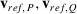are obtained using standard formulas for a point on a rigid body. The creepages are then obtained as the scaled velocity difference.

The new approach that is used for the curved reference works largely the same as this previous approach. The main difference is that the reference velocities are computed at all contact grid points, $$\mathbf{p} _{I}=[x_{I}, s_{I}, n_{Ir}]^{T}$$, $$\mathbf{q} _{I}=[x_{I}, s_{I}, n_{Iw}]^{T}$$, sampling the wheel and rail surfaces, instead of using the origin only.

This grid-based approach provides further insights in the creepages $$\xi , \eta , \phi$$. Namely, we recover the results of the traditional approach with creepages when using the grid-based approach with modified grids $$\mathbf{p} ''_{I}= \mathbf{q} ''_{I}=[x_{I}, s_{I}, 0]^{T}$$. From this we learn that:

• for a wheel on a rail, the creep calculation amounts to approximating the wheel by a purely conical surface;

• for a ball on a plane, the creep calculation amounts to approximating the ball by a cylindrical surface.

These approximations may be understood by the analysis presented by Kalker [31, §1.7.3]. The lateral curvatures of ball, wheel and roller are represented in Kalker’s analysis in the conformity terms $$(-1)^{a+1} B^{(a)}(s)$$, which are ignored in the simplified form of Eq. (15). The traditional creep calculation may thus be improved upon by retaining the conformity terms.

### Step 2.b’, Introducing the mid-plane correction

A second extension to the rigid slip is that the normal and longitudinal directions are redefined temporarily to account for the surface inclination in longitudinal direction:

\begin{aligned} & {} \mathbf{v} _{mid} = \frac{1}{2} \left ( \frac{ \mathbf{v} _{P} }{ \| \mathbf{v} _{P} \| } + \frac{ \mathbf{v} _{Q} }{ \| \mathbf{v} _{Q} \| } \right ), \end{aligned}
(20a)
\begin{aligned} & {} \mathbf{t} '_{x} = - \frac{ \mathbf{v} _{mid}}{ \| \mathbf{v} _{mid} \| }, \;\; c = ( \mathbf{t} '_{x}, \mathbf{t} _{x} ) , \;\; s = ( \mathbf{t} '_{x}, \mathbf{n} ) , \end{aligned}
(20b)
\begin{aligned} & {} \mathbf{t} '_{x} = \left [ \textstyle\begin{array}{c} c \\ 0 \\ s \end{array}\displaystyle \right ], \;\; \mathbf{n} ' = \left [ \textstyle\begin{array}{c} -s \\ 0 \\ c \end{array}\displaystyle \right ], \;\; \mathbf{R} = \left [ \textstyle\begin{array}{c@{\quad }c@{\quad }c} \ c & \ 0 & -s \\ \ 0 & \ 1 & \ 0 \\ \ s & \ 0 & \ c \end{array}\displaystyle \right ] , \end{aligned}
(20c)
\begin{aligned} & {} \mathbf{v} '_{P} = \mathbf{R} ^{T} \mathbf{v} _{P} , \;\; \mathbf{v} '_{Q} = \mathbf{R} ^{T} \mathbf{v} _{Q} . \end{aligned}
(20d)

These equations are illustrated in Fig. 9. They are obtained using $$\mathbf{t} _{x}, \mathbf{n}$$ as the global system, making $$\mathbf{t} '_{x}, \mathbf{n} '$$ the local system and making (20d) to-local operations. The rigid slip is then computed in the temporary coordinate system, e.g. $$w_{x}=v_{Px'}-v_{Qx'}$$, as illustrated in Fig. 9.

This temporary change compensates an artificial effect that comes from using a prismatic curved reference surface, linearly extruded in the rolling direction. This effect is illustrated in Fig. 10: in a contact-following coordinate system that is moving with velocity $$V$$, the longitudinal velocities show up as $$v_{Px}\equiv -V$$ and $$v_{Qx}= -V\cos (\theta )$$. This introduces rigid slip $$w_{x}=-V(1-\cos (\theta ))$$, non-zero when there is a difference in surface inclination. Equation (20b) puts the tangent direction $$\mathbf{t} '_{x}$$ half-way between $$\mathbf{v} _{P}$$ and $$\mathbf{v} _{Q}$$. Using this we get $$v_{Px'}=v_{Qx'}=-V \cos (\theta /2)$$ and get no rigid slip anymore in the situation of Fig. 10.

This unwanted rigid slip is described by the terms $$\frac{1}{2} R_{ax}^{-1} x^{2}$$ in Kalker’s analysis. Kalker stated that these terms are very small and can hence be neglected [31, p. 42]. Here we argue differently: the terms are not negligibly small by themselves, but will cancel each other when measured in normal rather than vertical direction.

### Step 3.c’, Aggregating tractions into resultant forces

For the aggregation of total forces, we consider the tractions $$\mathbf{p} = \mathbf{p} ^{(1)}$$ acting on the grid locations $$\mathbf{x} _{I}$$ and in the coordinate directions of the curved reference surface. They are rotated to a common, Cartesian coordinate system by a generic curved-to-Cartesian operation. This can be the local coordinate system introduced for planar contact analysis, adhering to the existing flow of computations, reusing the existing code where possible. The rotated tractions may be denoted as $$p_{I\bar{n}} = p_{\bar{n}}(\tilde{x}_{I},\tilde{s}_{I})$$ for a traction in overall $$\bar{n}$$-direction at grid point $$I$$ of the curved reference surface.

The resultant forces acting on the upper body, the rail, are obtained by integration:

$$F_{\bar{n}}^{(1)} = \int _{\tilde{C}} p_{\bar{n}}(\tilde{x},\tilde{s}) \, d\tilde{x}\, d\tilde{s} \;\;=\;\; \sum _{I} p_{I\bar{n}} \,\delta x \,\delta s ,$$
(21)

likewise for $$F_{\bar{x}}$$ and $$F_{\bar{s}}$$. These forces act in the positive directions of the (planar) contact reference coordinates. The forces exerted on the wheel are obtained by “action = –reaction”.

The moments of the tractions $$\mathbf{p}$$ with respect to the contact reference position are computed similarly, using the same transformation. The contribution of a single point is obtained from the lever arm times surface tractions:

$$\mathbf{M} = \mathbf{x} \times \mathbf{p} = \left [ \textstyle\begin{array}{c} \bar{x} \\ \bar{s} \\ \bar{n} \end{array}\displaystyle \right ] \times \left [ \textstyle\begin{array}{c} p_{\bar{x}} \\ p_{\bar{s}} \\ p_{\bar{n}} \end{array}\displaystyle \right ] = \left [ \textstyle\begin{array}{c} \bar{s} \,p_{\bar{n}} - \bar{n} \,p_{\bar{s}} \\ \bar{n} \,p_{\bar{x}} - \bar{x} \,p_{\bar{n}} \\ \bar{x} \,p_{\bar{s}} - \bar{s} \,p_{\bar{x}} \end{array}\displaystyle \right ] .$$
(22)

The total moments are then obtained by integration over the contact area, e.g.

$$M_{\bar{x}}^{(1)} \;=\; \int _{\tilde{C}} \left ( \bar{s} \cdot p_{ \bar{n}}(\tilde{x},\tilde{s}) - \bar{n} \cdot p_{\bar{s}}(\tilde{x}, \tilde{s}) \right ) \, d\tilde{x}\, d\tilde{s} \;=\; \sum _{I} \left ( \bar{s}_{I} \, p_{I\bar{n}} - \bar{n}_{I} \, p_{I\bar{s}} \right ) \delta x\,\delta s$$
(23)

The components $$M_{\bar{x}}$$ and $$M_{\bar{s}}$$ are zero when using planar contact ($$\bar{n}\equiv 0$$) with a symmetric pressure distribution. The component $$M_{\bar{n}}$$ is called the spin moment.

To aggregate the total forces and moments of different contact patches requires that they are brought together in a common coordinate system. Obvious choices for actions on the rail are to use orientations of rail or track systems, while forces and moments on the wheel are interpreted more easily in the wheelset or wheel coordinate frames. The fictitious location of the aggregate forces may be shifted by adding compensating moments. For contact patch $$i$$, we can compute the equivalent force and moment acting at the rail marker as

\begin{aligned} & {} \mathbf{F} _{i(tr)} = \mathbf{R} _{i(tr)} \mathbf{F} _{i(cp)} , \;\; \mathbf{M} _{i@cp(tr)} = \mathbf{R} _{i(tr)} \mathbf{M} _{i@cp(cp)}, \end{aligned}
(24a)
\begin{aligned} & {} \mathbf{M} _{i@rr(tr)} = \mathbf{M} _{i@cp(tr)} + \left ( \mathbf{x} _{i(tr)} - \mathbf{x} _{rr(tr)}\right ) \times \mathbf{F} _{i(tr)} . \end{aligned}
(24b)

The total forces can be shifted similarly to any point $$P$$. The resultant couple can be made zero if the total force and moment vectors are perpendicular to one another. Else, we can split the moment in parallel and perpendicular parts and annihilate the latter by shifting: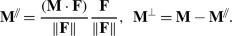(25)

When a force $$\mathbf{F}$$ is shifted from its original location $$\mathbf{0}$$ to $$\mathbf{x} _{av}$$, then this shift is taken into account in the moment by an additional term $$\mathbf{x} _{av}\times \mathbf{F}$$. Using the vector triple product,

$$\mathbf{a} \times ( \mathbf{b} \times \mathbf{c} ) = ( \mathbf{a} \cdot \mathbf{c} ) \mathbf{b} - ( \mathbf{a} \cdot \mathbf{b} ) \mathbf{c} ,$$
(26)

we may eliminate $$\mathbf{M} ^{\perp }$$ as follows:

\begin{aligned} {} \mathbf{x} _{av} \times \mathbf{F} = - \mathbf{M} ^{\perp } \rightarrow & {} \mathbf{F} \times ( \mathbf{x} _{av} \times \mathbf{F} ) = \mathbf{F} \times (- \mathbf{M} ^{\perp }) \end{aligned}
(27a)
\begin{aligned} \rightarrow & ( \mathbf{F} \cdot \mathbf{F} ) \mathbf{x} _{av} - ( \mathbf{F} \cdot \mathbf{x} _{av}) \mathbf{F} = - \mathbf{F} \times \mathbf{M} ^{\perp } \end{aligned}
(27b)
\begin{aligned} \stackrel{( \mathbf{F} \cdot \mathbf{x} _{av})=0}{\rightarrow } & {} \mathbf{x} _{av} = - \frac{ \mathbf{F} \times \mathbf{M} ^{\perp } }{ ( \mathbf{F} \cdot \mathbf{F} ) } = \frac{ \mathbf{M} ^{\perp } \times \mathbf{F} }{ ( \mathbf{F} \cdot \mathbf{F} ) } = \frac{ \mathbf{M} \times \mathbf{F} }{ ( \mathbf{F} \cdot \mathbf{F} ) } . \end{aligned}
(27c)

The location $$\mathbf{x} _{av}$$ may be placed at the rail surface by shifting along the force direction $$\mathbf{F} /\| \mathbf{F} \|$$, with no effect on the total force and moment. This involves the intersection of a spline curve with a straight line, which is solved by an iterative calculation. This finally defines an average location where the total forces may be conceived, collapsing the surface tractions with the smallest possible moment. This reduces the errors when moments are neglected in a multibody simulation.

## Results

This section presents numerical results computed with the new method and accompanying results of planar contact methods as listed in Table 1. All results are computed using the CONTACT program, version 20.2 , using stand-alone contact analysis. Two sets of test-cases are presented, using worn profiles obtained from one North American railroad in Sect. 4.1, and using a bigger collection of measured wheel profiles, obtained from another North American railroad, in Sect. 4.2.

The first area of application for the curved reference lies in the study of conformal and two–point wheel–rail contacts. This is illustrated in Sect. 4.1, with Sect. 4.1.1 discussing how discontinuities in the resultant forces may be avoided using the new method, and Sect. 4.1.2 addressing the refined calculation of the rigid slip velocities and their effect on the creep forces. The second application concerns the benchmarking and tuning of planar contact approaches. This is shown in Sect. 4.2, presenting a statistical evaluation of planar methods compared to the new curved reference approach.

### Two-point and conformal wheel/rail contacts

An interesting case for two–point and conformal contacts was obtained thanks to Dr. Kevin Oldknow [Simon Fraser Univ., pers.comm]. This concerns a small collection of wheel and rail profiles, gathered during field work for a North American heavy haul railway in 2005 and recognized as test-cases with conforming shapes. Rail profiles were measured for three different curves with nominal radii $$257~\mbox{m}$$, $$282~\mbox{m}$$, and $$388~\mbox{m}$$. Wheel profiles were collected from revenue service wagons with standard North American three-piece bogies and a gross load of 130 tonnes. Profiles were measured using MiniProf wheel profilometers for wheels with low, mid and high mileage.

Here we focus on the $$257~\mbox{m}$$ radius curve interacting with worn wheels with mid and high mileage. Figure 11 shows how the profiles of the high rail and of the high-mileage wheel produce a distinctly conformal contact. Test-cases for the contact analysis for the high rail are created using representative values obtained from geometry and kinematic data: nominal wheel load $$160~\mbox{kN}$$ for a loaded wagon, angle of attack $$\psi =5~\mbox{mrad}$$, roll angle $$\phi =-1.3~\mbox{mrad}$$. Full flange contact occurs when the wheel profile is shifted by $$y_{ws}=14.85~\text{mm}$$ from the nominal position, owing to the reduced flange thickness $$S_{d}=30.9~\text{mm}$$ and wear of the rail. The coefficient of friction is set to $$\mu =0.35$$ at the top of the rail (surface inclination $$\alpha \ge -20^{\circ }$$) and $$\mu =0.20$$ at the gauge face ($$\alpha \le -30^{\circ }$$) with a linear transition in between, modelling the use of flange lubrication.

#### Combined computation of contact patches

A difficult feature for the planar contact approach lies in the separate or combined computation of adjacent contact patches. This is illustrated in Figs. 12 and 13 for the planar contact method “icp-loc” of Table 1, which uses the initial contact position and the corresponding contact angle. The high-mileage wheel is considered at fixed velocities $$v=15~\mbox{m}/\mbox{s}, \omega _{ws}=-32.1~\text{rad}/\text{s}$$ (nominal rolling radius $$r_{nom}= 444~\text{mm}$$). One, two or three contact patches may be found in this configuration, at the wheel flange, the flange root, and the tread of the wheel, varying the vertical displacement $$z_{ws}$$ of the wheelset, at fixed lateral displacement $$y_{ws}=14.85~\text{mm}$$. The interpenetration areas on the flange root and tread merge when the wheel is moved down beyond $$z_{ws}=1.18~\text{mm}$$, such that two contact patches are computed from thereon (compare Figs. 12 (b) and (c)). This gives a drop of $$18.1~\mbox{kN}$$ in the vertical forces computed and an accompanying change in the lateral forces, increasing $$L/V$$ from 0.92 to 1.13, as shown in Fig. 13.

Two different effects occur when two contact patches are taken together in the planar contact approach:

1. 1.

The contact reference angle changes suddenly from two values for the separate patches to a single value when they are combined.

2. 2.

The contact pressures of the two patches suddenly start interacting with each other. This generally increases the computed elastic deformations, reducing the pressures needed at fixed interpenetration.

The effect of the contact angle can be lessened to some extent using an averaged value instead of the value sampled at the initial contact position. This works especially for the case of Fig. 12 (c) where patches on the flange root and tread of the wheel are combined. Bigger difficulties remain if the contact patch on the flange needs to be combined with the one on the flange root, due to the bigger changes in contact angle.

The effects of interactions are seen in Fig. 13 at $$z_{ws}\le 1.18~\text{mm}$$. As the contact is nearly flat within each of the three contact patches, close correspondence could be expected between the planar and conformal approaches. Yet the conformal method finds considerably lower pressures and forces compared to the planar method. This is the result of taking the interactions between different patches into account instead of neglecting them. This is achieved by “greedy” combination of patches in the conformal approach. Interpenetration regions are combined there that lie less than $$d_{sep}=30~\text{mm}$$ from each other, resulting in a single patch in Figs. 12 (d)–(f).

These discontinuities in the total forces pose a trade-off between reliability and robustness for the planar contact approach. Different strategies are followed by vehicle dynamics softwares. VAMPIRE, for instance, tends to avoid jumps in the results by supporting at most one contact on the tread of the wheel and at most one at the flange, at the expense of the trustworthiness of the results in more challenging situations. SIMPACK and Universal Mechanism, on the other hand, support multiple contact patches, appearing, disappearing, and merging as the simulation evolves. This goes at the expense of some robustness and performance, using time step refinement and permitting some irregularities in the results when jumps occur.

The benefit of the conformal contact approach is to provide smoothness by using the local contact angles, for the undeformed distance as well as the rigid slip computation. Jumps regarding the contact interaction can then be mitigated using greedy combination of patches, or using a blending approach in which the interaction is gradually turned on when contact patches get closer to one another .

#### Effects of the rigid slip computation

The effects of different computational methods on the frictional forces are examined with a test for the mid-mileage wheel. The wheelset is placed at lateral position $$y_{ws}=10.65~\text{mm}$$ ($$S_{d}=36.3~\mbox{mm}$$) and roll angle $$\phi _{ws}=1.3~\mbox{mrad}$$, pressed down to a vertical load $$F_{z(tr)}=160~\mbox{kN}$$. The forward velocity and yaw angle are kept fixed at $$v=15~\mbox{m}/\mbox{s}$$ and $$\psi _{ws}=5~\mbox{mrad}$$. The angular velocity $$\omega _{ws}$$ is varied in a wide range to explore how this affects the creep forces. Results are shown in Figs. 1416 for the methods icp-loc and wgt-loc-2 of Table 1.

Figure 14 shows considerable differences between the creep forces computed using different methods. A first origin for this lies in the coefficient of friction employed in the different calculations. In the planar contact methods, a constant value is used that is determined on the basis of the contact reference angle. The initial contact method finds contact angle $$\delta =-32.6^{\circ }$$, by which the case is considered as flange contact with $$\mu =0.20$$. The weighted center method, on the other hand, finds $$\delta =-18.6^{\circ }$$ and sets $$\mu =0.35$$ for tread contact. The conformal contact method, using a variable coefficient of friction, on the basis of the actual inclination of the curved reference surface, produces $$\mu =0.2$$ on the left side and $$\mu =0.35$$ on the right side of the contact area.

Looking in detail, we find that the different coefficients of friction do not fully explain the different shapes of the curves: a slower transition from negative to positive $$F_{x}$$ for the initial contact method, and attaining the lowest lateral creep-force values at different angular velocities $$\omega _{ws}$$. These differences are analyzed further by considering one specific case, at $$\omega _{ws}=-33.7~\text{rad}/\text{s}$$, in Figs. 1517.

The slower transition found for the initial contact method is attributed mainly to the rigid slips $$[w_{x}, w_{y}]^{T}$$ that are used (Fig. 16). These are understood with the aid of the contact situations as illustrated in Fig. 17. The curved reference method computes rigid slip velocities based on the actual wheel and rail surface shapes, that is, using the local kinematics at each surface position. The planar methods approximate this using the kinematics at a single position, i.e. the contact reference marker. At their respective contact reference positions, both planar contact methods thereby produce the same rigid slip as the conformal method. Yet the methods differ in the rigid slips computed at other positions. Using Eq. (15), these rigid slips vary linearly in the planar methods. The coefficient $$\phi$$ is bigger for the initial contact method corresponding to the bigger contact angle. For the conformal method, the rigid slips vary more rapidly on the left side, and slower on the right, in proportion to the inclination of the contact reference surface. This corresponds to the conformity terms $$B^{(a)}(s)$$ as discussed in Sect. 3.5.

A similar effect plays a role in the lateral creep forces $$F_{s}$$. Further, the coefficient of friction plays a role in the results for the weighted center method, with bigger stresses $$p_{y}<0$$ in the range $$-10~\mbox{mm}\le y_{c}\le -5~\text{mm}$$ (Fig. 15). Thirdly, we find that the aggregate results plotted for the curved reference method are affected by the contact pressures. This concerns the force $$F_{\bar{s}}$$ for the contact reference orientation, as discussed in Sect. 3.7. Depending on the actual orientation of the contact pressures, positive contributions are found on the left and negative on the right of the reference position. This may be perceived as a downside of the curved reference surface: the results are not interpreted as easily as for the planar contact approach.

### Statistical evaluation of planar contact approaches

The results shown above suggest that the initial contact approach is more susceptible to jumps in forces than the weighted center approach, and that it may be better to use an averaged contact angle than sampling the angle at the reference position. So far, however, this is just anecdotal evidence, with no indication on the generality of the results. Here we present statistics for a large number of cases to get more balanced information.

#### Data-set for freight cars with worn profiles

Data are obtained from project work for CSX, a US class I railroad, performed by Sentient Science, NRC Canada, and Vtech CMCC on rail life and rail life extension. The overall simulation approach starts by selecting a curve and collecting traffic data: the number of trains, types of wagons, loads, speeds, wheel profiles, as well as track data, e.g. geometry, friction management practices. These data are analyzed and classified, e.g. distinguishing different quality levels . Loading ensembles are then defined that represent the actual situation, maintaining the ranges and variability of input parameters, however, with a much reduced number of loadings. Simulations with VAMPIRE are used to assess the vehicle dynamics in steady curving, using VAMPIRE’s internal contact algorithms. The contact situation is then analyzed anew, in more detail, using CONTACT, and the resulting contact stresses are fed into Sentient’s calculation of wear and grinding. This is repeated many times in a cycle, representing an overall duration of 25 years or so, until the rail profile is worn down to a predefined threshold and the life is exhausted.

Here we consider the data obtained for a 5 degree right hand curve (radius $$349~\mbox{m}$$), near Cartersville, GA. A thousand freight cars are simulated, a mixture of flat, hopper and tank cars, with heavily worn wheel profiles, according to the 20% of wheels with the lowest quality index. Speeds vary between 7 and $$40~\mbox{mph}$$ (11 to $$64~\mbox{km}/\mbox{h}$$), and weights between $$50\,000$$ and $$300\,000~\mbox{lbs}$$ (222 to $$1334~\mbox{kN}$$) for empty and full wagons. Two axles are considered for each car, for the leading bogie. Four coefficients of friction are set for each axle, two for each wheel, taken from normal distributions with mean value $$\bar{\mu }=0.35$$ and standard deviation $$\sigma _{\mu }= 0.16$$ at the top of the rail (surface inclination $$\alpha \le 20^{\circ }$$) and $$\bar{\mu }=0.20, \sigma _{\mu }=0.0167$$ at the gauge face ($$\alpha \ge 30^{\circ }$$). Two rail profiles are considered: tentative new profiles for the high and low rails of the curve.

CONTACT calculations are performed once as a post-processing step after each VAMPIRE simulation. This gives a considerable speed up compared to full integration of CONTACT in the multibody simulation, owing to the fast heuristic contact algorithms implemented in VAMPIRE, executed many times to find the steady curving situation. Calculations with CONTACT are then done with wheelset kinematics held fixed as computed by VAMPIRE, including axle positions, orientations and velocities. One exception concerns the vertical position. Each wheel is raised or lowered as needed to maintain the vertical force $$F_{z(tr)}$$ obtained from VAMPIRE. This addresses the differences in the relation between position and vertical force between CONTACT and VAMPIRE’s contact methods. Agreement in the positions is considered much less important than agreement in the forces, here, because the positions have no direct influence on the wear calculation, whereas the forces govern the stresses that do have a major effect on the wear calculation.

#### Detailed inspection for wheel 2446

Results are computed for all wheels using different methods and compared in different ways. The wheels that showed the biggest differences were investigated further. Figures 18 and 19 show the results for wheel 2446, which are particularly insightful on how the different strategies work out for the contact reference point and reference angle.

The wheel profile that is used exhibits some grooves and ridges across the profile. This manifests itself as oscillations in the surface inclination (Fig. 18, left) and gives rise to disjoint valleys in the undeformed distance function (right). At a vertical force of $$152.7~\mbox{kN}$$, these valleys are contained in one interpenetration area with two local minimum values. This results in two actual contact areas with nearly elliptical shapes, with some interactions between the two patches.

Figure 19 shows the contact planes and markers used with the resulting pressure distributions. This shows clearly how the initial contact method places the contact reference at the deepest bump (b)–(c), while the weighted center method goes somewhere in the middle (e)–(f). Next, the effect of an averaged angle is seen clearly comparing pictures (b) and (c) for the initial contact method. The weighted center + angle method (e) uses the same angle as (b) but raises the contact plane slightly due to the different reference position. This gives a slight difference in rolling radius between the weighted center and initial contact methods when using the averaged contact angle.

Figure 18 (left) shows the inclinations of various tangent planes using dashed and dotted lines. The dashed line for the weighted averaging methods approximates the average value for the curve for the curved reference surface. The approximation used by the icp + local method is rather poor because it samples the actual inclinations away from the center. The value used by wgt(lin) + local appears to underestimate the averaged value. This is explained by the convex shape of the actual curve, which is a typical feature of the rail gauge corner.

Table 2 shows the total forces obtained from different methods. For icp + local we see that over-estimating the contact angle results in a bigger lateral creep force $$F_{s}$$, reducing $$F_{x}$$. Furthermore, the contact angle lets $$F_{n}$$ contribute stronger to $$F_{y}$$. For wgt + local, under-estimating the contact angle, $$F_{s}$$ is reduced slightly compared to wgt + angle while $$F_{x}$$ is increased. The main change comes again from the propagation of $$F_{n}$$ on $$F_{y}$$. The wgt + angle and icp + angle methods seem to perform a little worse in $$F_{x}$$ and $$F_{s}$$ but compensate through the $$F_{n}$$ contribution. Squared weights improve the contact angle and the ultimate correspondence of the results.

#### Evaluation of methods

Many different planar contact methods can be constructed using different strategies for defining the contact reference position and reference angle. The question is: which of these methods comes closest to the true contact situation? This is assessed for the methods as listed in Table 1 with the curved reference method as referee, i.e. the best method currently available at our disposition.

The results of different calculations are compared mainly through the signed derailment coefficients $$L/V$$. These are sensitive to the contact angle and contact location, while ignoring the different weights of different wagons. Figure 20 shows the comparison for a number of methods, with the wheels sorted by the $$L/V$$ values computed by the curved reference method. This shows that there is a bigger spread in the computed results for wheels with negative $$L/V$$ values. These are mostly wheels on the left side, the high rail, where we find multiple contacts and contacts with bigger contact angles.

Table 3 shows the statistics of the differences of each method compared to the curved reference method. This takes each line of Fig. 20 in turn, computes the difference for each wheel compared to the reference method, and then computes the mean value, mean of absolute values, root-mean-square, and maximum absolute values.

The first line of Table 3 concerns the comparison of different variants of the curved reference method, using different ways to define the reference position. This is used as an anchor for the curved reference surface and its grid discretization. The table shows that there is a slight dependence on the way how the contact reference position is selected. These differences diminish when the grid is refined.

The second part of the table shows results for the weighted center method. This appears to give somewhat biased results (nonzero mean difference), when sampling the contact angle at the reference position. This is seen in Fig. 20 as well, with more yellow above than below the results for the curved reference method. This comes from the typical shapes of rail profiles, with low curvature at the top of the rail and curvature increasing towards the gauge corner. The weighted center method places the reference position centered in the contact patch, on average. The surface inclination that is found there is then typically a little lower than the average inclination over the width of the contact.

The third part of the table concerns the initial contact method. Here we found 7 wheels with $$L/V$$-values below −1.0, related to big contact angles at the flange of the wheel. These are filtered out to avoid their big influence on the statistics. After this, we still find nearly twice bigger differences than for the weighted center method with sampled contact angles. These differences are removed when switching to an averaged contact angle. We conclude that the contact angle is the most sensitive parameter in the planar contact approach. Weighted averaging appears to give better values than local sampling, and the squared gaps of Eq. (14) work a little better than the linear gaps used in Eq. (13).

#### Performance

Considering the performance, each of the methods needs about $$450~\mbox{s}$$ on a commodity laptop for the computations for $$4\,000$$ wheels. About $$20~\mbox{s}$$ is spent on the geometrical analysis, $$60~\mbox{s}$$ on the normal contact problem, $$310~\mbox{s}$$ on the tangential problem, and $$60~\mbox{s}$$ on in- and output. In this time, each wheel is analyzed $$4\text{--}5$$ times to satisfy the requested vertical force, using about $$25~\mbox{ms}$$ per case with prescribed positions. The resolution used is $$\delta x= \delta s=0.5~\text{mm}$$, by which $$60\text{--}1120$$ elements end up in contact, with an average of 325. The main difference between the curved reference and planar methods lies in the geometrical part. The curved reference method needs $$30\%$$ more time there, corresponding to an increase of about $$1\%$$ of the total time.

## Conclusions and discussion

This paper addressed the role of the tangent plane in the planar contact approach –defining the normal and tangential directions needed for the undeformed distance, creepage, pressures, and slip– and discussed the complications in its definition, prior to the calculations, and using a single inclination to approximate the entire contact. The curved reference surface is put forward as an alternative backbone for detailed wheel/rail contact computations. This builds on the generalized coordinates $$[x,s,n]^{T}$$ introduced for conformal contact situations that provide different normal and tangential directions at different locations. This facilitates the use of local kinematics instead of approximate, global values, by-passing the creepages, evaluating the rigid slip velocities in a more detailed way. The steps are described by which the curved reference is constructed, using generic facilities for markers, grids, and interpolations, enabling the conformal method to be used in a fully automated way.

A main characteristic of the curved reference surface that is used in CONTACT is that it uses a local construction. Separate curved reference grids are produced for different contact patches, to avoid the complications that arise in global curvilinear coordinates for surfaces that separate by a bigger distance and then come back together. A beneficial effect of this choice is that it permits re-using the steps for planar wheel/rail contact solution with relatively minor extension.

The computation of the curved reference surface is based on the chord distances $$s_{r}$$ and $$s_{w}$$ along the wheel and rail profiles. Earlier calculations on this are extended for the 3-d contact search procedures used in CONTACT, including yaw. The surface is represented by a planar curve in the $$Oyz$$-plane of the track frame, which is extruded in the direction of rolling. This makes the curved reference easier to understand and work with because orientations like the local normal can be described using a single rotation. This simplified view creates an artifact in the rigid slip calculation that is resolved locally using the so-called midplane correction.

One application of the curved reference surface is to provide reference solutions for the evaluation of simpler contact approaches. This is worked out for the tangent plane used in the planar contact approach. A data-set is used for a thousand freight cars with heavily worn wheel profiles, with statistical evaluation. The tangent plane’s inclination appears to be the most influential aspect, which needs to approximate the average value over the contact region. After this, the reference position for the local coordinate system can be defined equally well with the initial contact position or using weighted averaging.

The planar contact approach seems to give quite acceptable results in most cases, but may break down in particular circumstances. This happens mainly for measured, worn profiles where multiple contact patches are forming and growing together. Discontinuities are found in the resultant contact forces, originating from sudden changes in the contact reference angle and in the interactions between different contact patches. In such cases, the planar contact approach is restricted by the use of a constant inclination for the tangent plane, while the curved reference accommodates variations in inclination naturally. This permits for more smooth and robust contacts in multibody simulation.

The benefits of the curved reference may be exploited also in heuristic contact approaches, for instance using the Kik–Piotrowski method in combination with Fastsim. Discontinuities may be reduced there as well, and creepages may be avoided using the more detailed rigid slip computation. This is an interesting topic for further study. Another way forward is to improve the planar contact approach with the aid of the curved reference surface. One could improve the calculation of creepages for instance by adding a conformity term. A third direction for future work concerns truly conformal contacts: assessing the consequences of our simplifications, extending the influence coefficients used beyond the halfspace approach, and comparing the results to those obtained from finite-element calculations. A final challenge remains to get better understanding of the coefficient of friction: how it comes about, and how influential factors like fluids and third body layers can be included in our modeling.